TensorSpace.jsTensorSpace Converter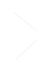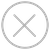TensorSpace Layer 具有两种状态：open（展开） 和 close（闭合），默认为 “close”。我们可以在模型初始化之前 设置 Layer 的初始状态，也可以在模型初始化后 改变 Layer 的状态

``````let model = new TSP.models.Sequential( container, {
// 或 "close"
layerInitStatus: "open"
} );``````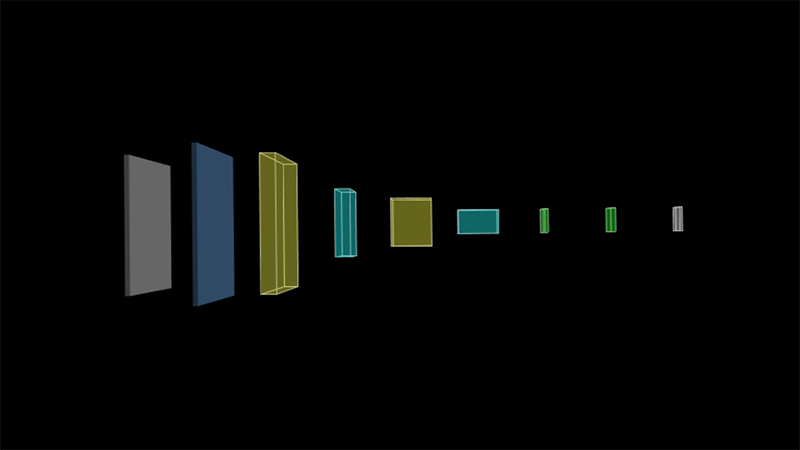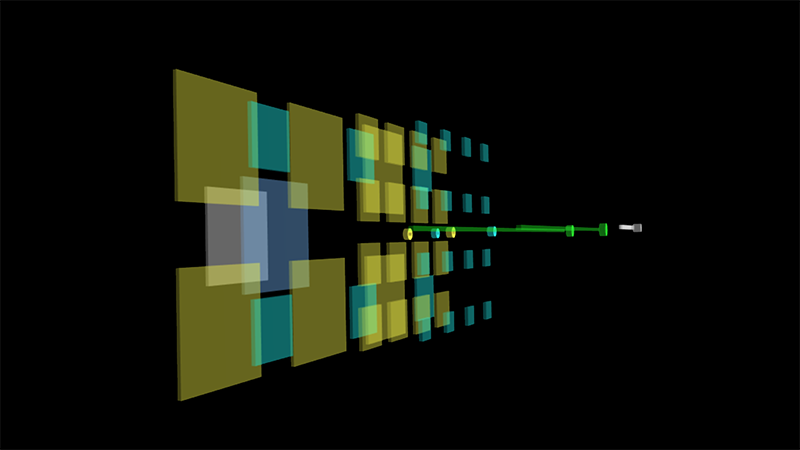``````let conv2dLayer = new TSP.layers.Conv2d({
// 或 close
initStatus: "open"
});``````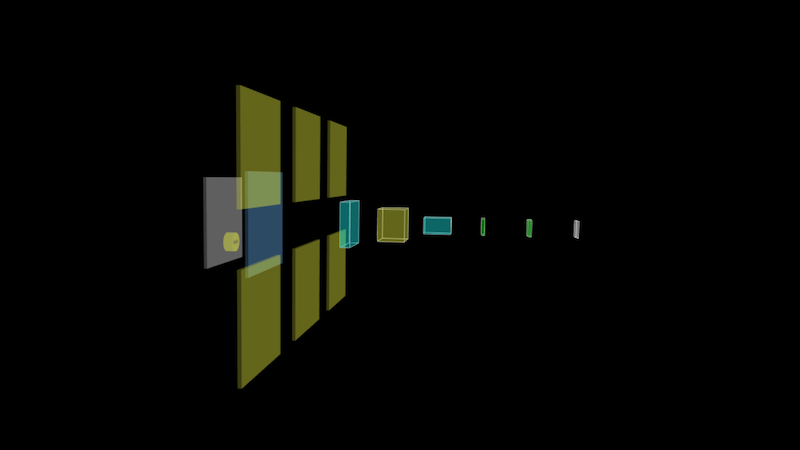3D场景中点击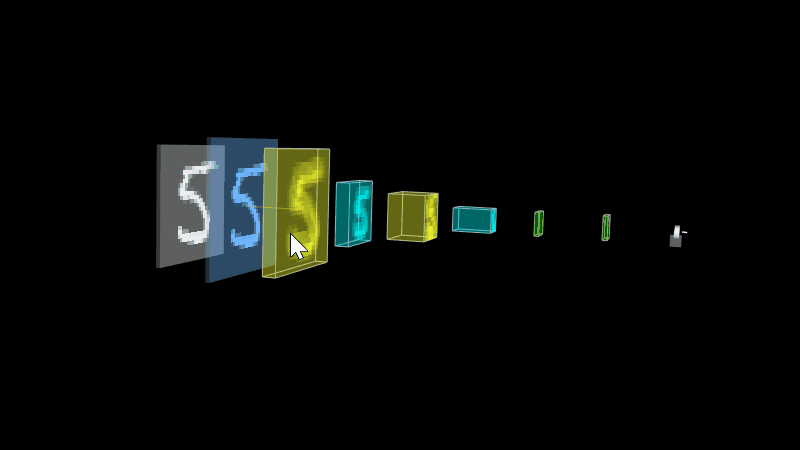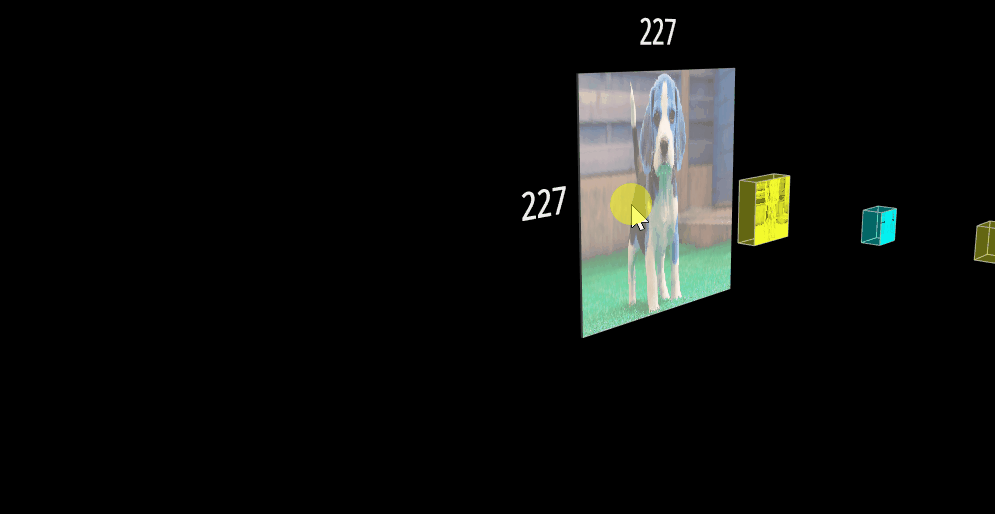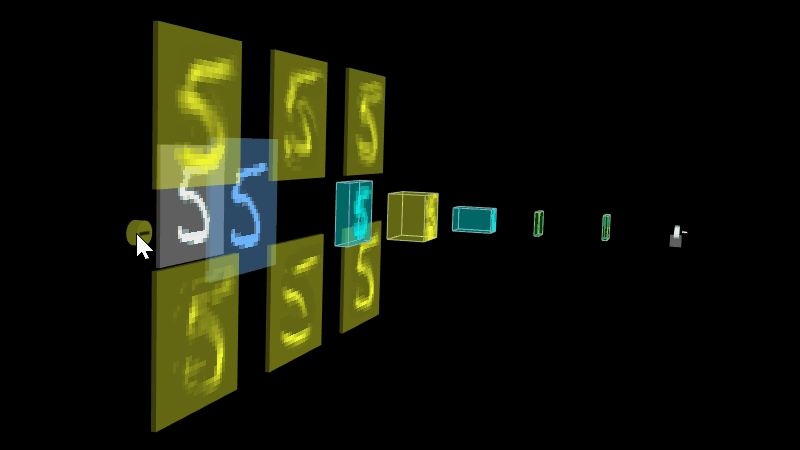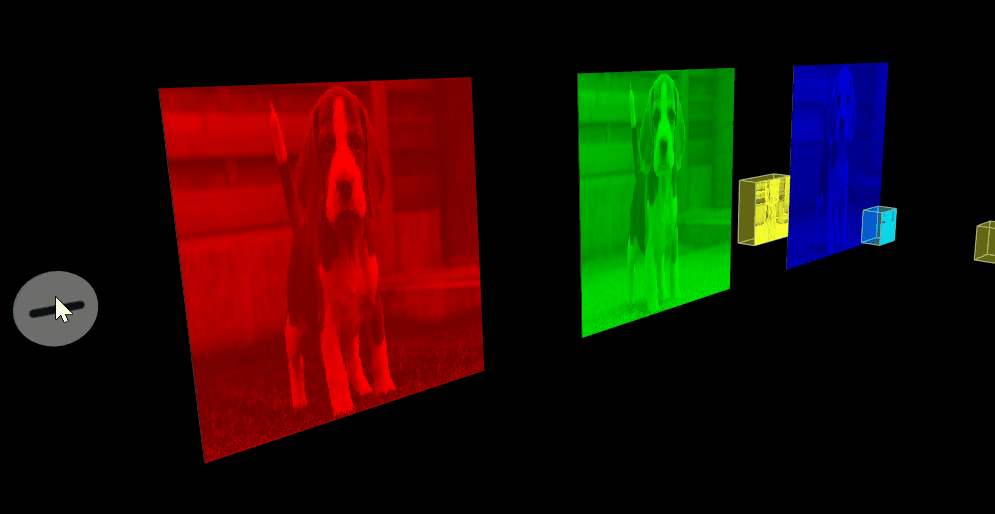``````let conv2dLayer = new TSP.layers.Conv2d( config );

// ... 给模型添加更多的layer。

model.init();

// 调用openLayer API来展开layer。
conv2dLayer.openLayer();``````

``````let conv2dLayer = new TSP.layers.Conv2d( config );